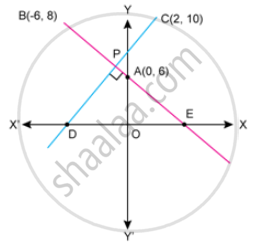Advertisement Remove all ads

# In the Given Figure. Line Ab Meets Y-axis at Point A. Line Through C(2,10) and D Intersects Line Ab at Right Angle at Poiunt R Find (1) Equation of Line Ab (2) Equation of Line Cd - Mathematics

Sum

In the given figure. line AB meets y-axis at point A. Line through C(2,10) and D intersects line AB at right angle at poiunt R find

(1) equation of line AB

(2) equation of line CD

(3) Co-ordinates of point E and DAdvertisement Remove all ads

#### Solution

(1) "Slope of line AB "=m=(8-6)/(-6-0)=2/-6=-1/3

The y-interoept of the line Ab is 6.

Thus , the equation of th given line is given by the slope -intercept from y=mx+c

i.e. y=-1/3xx+6

i.e. 3y=-x+18

i.e. x+3y=18, Which is the required equation.

(2) Since Ab and CD intersect at right angles,

"slope"_(AB)xx "slope"_(CD)=-1

⇒ -1/3xx "slope"_(CD)=-1

⇒ Slope_(CD)=3

U sing the slope-point from, the equation of CD is given by

y-y_1=m(x-x_1)

i.e. y-10=3(x-2)

i.e. y-10=3x-6

i.e. 3x-y+4=0 Which is the required equation of line CD.

(3) Since point E satisfies the equation Of AB, and the y-coordinate of E is 0, We can find the x-Coodinate of E.

x+3(0)=18

⇒ x=18

So, the coordinates of E are (18,0).

Now, since point D satisfies the equation Of CD, and the y-coordinate of D is 0, We can find the x-coordinate Of D.

3x-(0)+4=0

⇒3x=-4

⇒x=-4/3

So, the coordinates of D are (-4/3,0)

Concept: General Equation of a Line
Is there an error in this question or solution?
Advertisement Remove all ads

#### APPEARS IN

Selina Concise Maths Class 10 ICSE
Chapter 14 Equation of a Line
Exercise 14 (E) | Q 34 | Page 204
Advertisement Remove all ads

#### Video TutorialsVIEW ALL 

Advertisement Remove all ads
Share
Notifications

View all notifications

Forgot password?
Course